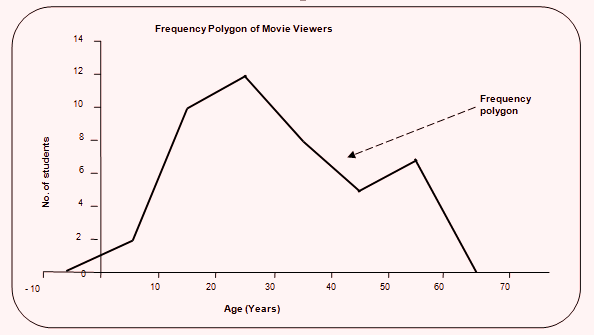# Frequency Polygon - Diagrammatic and Graphical Representation of Data | Economics Notes Grade XI

### Commerce Notes

☰   Related Articles

#### Scarcity And Choice

Concepts of Scarcity And Choice

#### Extra# Frequency Polygon - Diagrammatic and Graphical Representation of Data

Frequency Polygon
A frequency polygon is another device of graphic presentation of a frequency distribution. It is applicable in both discrete and continuous frequency distributions.

In case of discrete frequency distribution, frequency polygon is drawn by noting the frequency along the y-axis and the value of the variable along the x-axis. By joining the points on the graphs, we get frequency polygon. In the case of continuous frequency distributions, frequency polygon can be drawn in two ways:

a) Frequency polygon and histogram: Frequency polygon is drawn by joining the mid-points of the tops (upper horizontal sides) of the variable along the x-axis. By joining the points on the graph, we get frequency polygon. In the case of continuous frequency distributions, frequency polygon can be drawn in two ways:

Example:

 Age (Years) 0 – 10 10 – 20 20 – 30 30 – 40 40 – 50 50 – 60 No. of movie viewers 2 10 12 8 5 7b) Frequency polygon without histogram: Frequency polygon can be drawn without drawing a histogram. For this, the mid-values of the class intervals should be calculated. The mid-values are measured along the x-axis and the corresponding frequencies along the y-axis. By plotting and joining the points, frequency polygon is obtained. The end of the frequency polygon should be joined with zero frequencies, one at the beginning of the given classes and the other at the end of the classes. It should be remembered that the area covered by histogram should be equal to the area of the frequency polygon.

Example:

 Age (Years) Mid-value No. of movie viewers 0 – 10 5 2 10 – 20 15 10 20 – 30 25 12 30 – 40 35 8 40 – 50 45 5 50 – 60 55 7## Popular Subjects

Join with us on social media to see our updates on your feed.